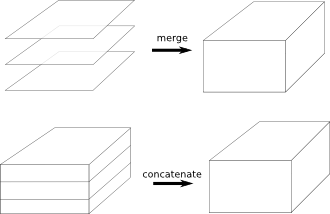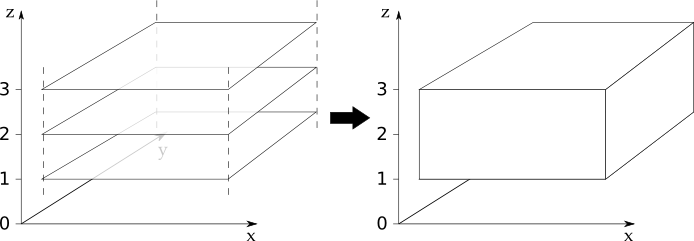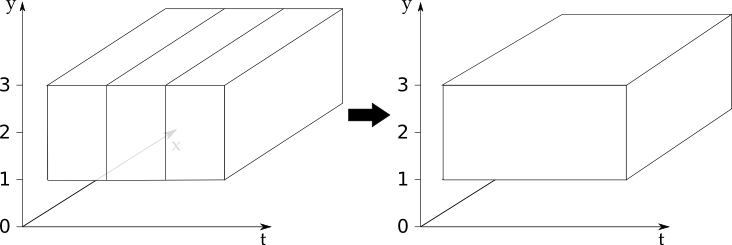# Merge and Concatenate¶

We saw in the Loading Iris Cubes chapter that Iris tries to load as few cubes as possible. This is done by collecting together multiple fields with a shared standard name (and other key metadata) into a single multidimensional cube. The processes that perform this behaviour in Iris are known as `merge` and `concatenate`.

This chapter describes the `merge` and `concatenate` processes; it explains why common issues occur when using them and gives advice on how prevent these issues from occurring.

Both `merge` and `concatenate` take multiple cubes as input and result in fewer cubes as output. The following diagram illustrates the two processes:There is one major difference between the `merge` and `concatenate` processes.

• The `merge` process combines multiple input cubes into a single resultant cube with new dimensions created from the scalar coordinate values of the input cubes.

• The `concatenate` process combines multiple input cubes into a single resultant cube with the same number of dimensions as the input cubes, but with the length of one or more dimensions extended by joining together sequential dimension coordinates.

Let’s imagine 28 individual cubes representing the temperature at a location `(y, x)`; one cube for each day of February. We can use `merge()` to combine the 28 `(y, x)` cubes into a single `(t, y, x)` cube, where the length of the `t` dimension is 28.

Now imagine 12 individual cubes representing daily temperature at a time and location `(t, y, x)`; one cube for each month in the year. We can use `concatenate()` to combine the 12 `(t, y, x)` cubes into a single `(t, y, x)` cube, where the length of the `t` dimension is now 365.

## Merge¶

We’ve seen that the `merge` process combines multiple input cubes into a single resultant cube with new dimensions created from the scalar coordinate values of the input cubes.

In order to construct new coordinates for the new dimensions, the `merge` process requires input cubes with scalar coordinates that can be combined together into monotonic sequences. The order of the input cubes does not affect the `merge` process.

The `merge` process can produce a cube that has more than one new dimension, if the scalar coordinate sequences form an orthogonal basis.

Important

The shape, metadata, attributes, coordinates, coordinates metadata, fill value and other aspects of the input cubes must be consistent across all of the input cubes.

The `merge` process will fail if these are not consistent. Such failures are covered in the Common Issues With Merge and Concatenate section.

The `merge` process can be accessed using two methods. The two methods are `merge()` and `merge_cube()`, which are described below.

### Using CubeList.merge¶

The `CubeList.merge` method operates on a list of cubes and returns a new `CubeList` containing the cubes that have been merged.

Let’s have a look at the `merge()` method in operation. In this example we have a list of three lateral (x, y) cubes in a variable called `cubes`, each with a scalar `z` coordinate of differing value. We can merge these cubes by stacking the scalar `z` coordinates to make a new `z` dimension coordinate:

```>>> print(cubes)
0: air_temperature / (kelvin)          (y: 4; x: 5)
1: air_temperature / (kelvin)          (y: 4; x: 5)
2: air_temperature / (kelvin)          (y: 4; x: 5)

>>> print(cubes)
air_temperature / (kelvin)          (y: 4; x: 5)
...
Scalar coordinates:
z                           1 meters
>>> print(cubes)
air_temperature / (kelvin)          (y: 4; x: 5)
...
Scalar coordinates:
z                           2 meters
>>> print(cubes)
air_temperature / (kelvin)          (y: 4; x: 5)
Dimension coordinates:
y                             x     -
x                             -     x
Scalar coordinates:
z                           3 meters

>>> print(cubes.merge())
0: air_temperature / (kelvin)          (z: 3; y: 4; x: 5)
```

The following diagram illustrates what has taken place in this example:The diagram illustrates that we have three input cubes of identical shape that stack on the `z` dimension. After merging our three input cubes we get a new `CubeList` containing one cube with a new `z` coordinate.

### Using CubeList.merge_cube¶

The `merge_cube()` method guarantees that exactly one cube will be returned as a result of merging the input cubes. If `merge_cube()` cannot fulfil this guarantee, a descriptive error will be raised providing details to help diagnose the differences between the input cubes. In contrast, the `merge()` method makes no check on the number of cubes returned.

To demonstrate the differences between `merge()` and `merge_cube()`, let’s return to our three cubes from the earlier merge example.

For the purposes of this example a `Conventions` attribute has been added to the first cube’s `attributes` dictionary. Remember that the attributes must be consistent across all cubes in order to merge into a single cube:

```>>> print(cubes)
0: air_temperature / (kelvin)          (y: 4; x: 5)
1: air_temperature / (kelvin)          (y: 4; x: 5)
2: air_temperature / (kelvin)          (y: 4; x: 5)

>>> print(cubes.attributes)
{'Conventions': 'CF-1.5'}
>>> print(cubes.attributes)
{}
>>> print(cubes.attributes)
{}

>>> print(cubes.merge())
0: air_temperature / (kelvin)          (y: 4; x: 5)
1: air_temperature / (kelvin)          (z: 2; y: 4; x: 5)

>>> print(cubes.merge_cube())
Traceback (most recent call last):
...
raise iris.exceptions.MergeError(msgs)
iris.exceptions.MergeError: failed to merge into a single cube.
cube.attributes keys differ: 'Conventions'
```

Note that `merge()` returns two cubes here. All the cubes that can be merged have been merged. Any cubes that can’t be merged are included unchanged in the returned `CubeList`. When `merge_cube()` is called on `cubes` it raises a descriptive error that highlights the difference in the `attributes` dictionaries. It is this difference that is preventing `cubes` being merged into a single cube. An example of fixing an issue like this can be found in the Common Issues With Merge and Concatenate section.

The CubeList’s `merge()` method is used internally by the three main Iris load functions introduced in Loading Iris Cubes. For file formats such as GRIB and PP, which store fields as many individual 2D arrays, Iris loading uses the `merge` process to produce a more intuitive higher dimensional cube of each phenomenon where possible.

Sometimes the `merge` process doesn’t behave as expected. In almost all cases this is due to the input cubes containing unexpected or inconsistent metadata. For this reason, a fourth Iris file loading function, `iris.load_raw()`, exists. The `load_raw()` function is intended as a diagnostic tool that can be used to load cubes from files without the `merge` process taking place. The return value of `iris.load_raw()` is always a `CubeList` instance. You can then call the `merge_cube()` method on this returned `CubeList` to help identify merge related load issues.

## Concatenate¶

We’ve seen that the `concatenate` process combines multiple input cubes into a single resultant cube with the same number of dimensions as the input cubes, but with the length of one or more dimensions extended by joining together sequential dimension coordinates.

In order to extend the dimensions lengths, the `concatenate` process requires input cubes with dimension coordinates that can be combined together into monotonic sequences. The order of the input cubes does not affect the `concatenate` process.

Important

The shape, metadata, attributes, coordinates, coordinates metadata, fill value and other aspects of the input cubes must be consistent across all of the input cubes.

The `concatenate` process will fail if these are not consistent. Such failures are covered in the Common Issues With Merge and Concatenate section.

The `concatenate` process can be accessed using two methods. The two methods are `concatenate()` and `concatenate_cube()`, which are described below.

### Using CubeList.concatenate¶

The `CubeList.concatenate` method operates on a list of cubes and returns a new `CubeList` containing the cubes that have been concatenated.

Let’s have a look at the `concatenate()` method in operation. In the example below we have three 3D `(t, y, x)` cubes whose `t` coordinates have sequentially increasing ranges. These cubes can be concatenated by combining the `t` coordinates of the input cubes to form a new cube with an extended `t` coordinate:

```>>> print(cubes)
0: air_temperature / (kelvin)          (t: 31; y: 3; x: 4)
1: air_temperature / (kelvin)          (t: 28; y: 3; x: 4)
2: air_temperature / (kelvin)          (t: 31; y: 3; x: 4)

>>> print(cubes.concatenate())
0: air_temperature / (kelvin)          (t: 90; y: 3; x: 4)
```

The following diagram illustrates what has taken place in this example:The diagram illustrates that we have three 3D input cubes that line up on the `t` dimension. After concatenating our three input cubes we get a new `CubeList` containing one cube with an extended `t` coordinate.

### Using CubeList.concatenate_cube¶

The `concatenate_cube()` method guarantees that exactly one cube will be returned as a result of concatenating the input cubes. If `concatenate_cube()` cannot fulfil this guarantee, a descriptive error will be raised providing details to help diagnose the differences between the input cubes. In contrast, the `concatenate()` method makes no check on the number of cubes returned.

To demonstrate the differences between `concatenate()` and `concatenate_cube()`, let’s return to our three cubes from the earlier concatenate example.

For the purposes of this example we’ll add a History attribute to the first cube’s `attributes` dictionary. Remember that the attributes must be consistent across all cubes in order to concatenate into a single cube:

```>>> print(cubes)
0: air_temperature / (kelvin)          (t: 31; y: 3; x: 4)
1: air_temperature / (kelvin)          (t: 28; y: 3; x: 4)
2: air_temperature / (kelvin)          (t: 31; y: 3; x: 4)

>>> print(cubes.attributes)
{'History': 'Created 2010-06-30'}
>>> print(cubes.attributes)
{}

>>> print(cubes.concatenate())
0: air_temperature / (kelvin)          (t: 31; y: 3; x: 4)
1: air_temperature / (kelvin)          (t: 59; y: 3; x: 4)
>>> print(cubes.concatenate_cube())
Traceback (most recent call last):
...
raise iris.exceptions.ConcatenateError(msgs)
iris.exceptions.ConcatenateError: failed to concatenate into a single cube.
Cube metadata differs for phenomenon: air_temperature
```

Note that `concatenate()` returns two cubes here. All the cubes that can be concatenated have been concatenated. Any cubes that can’t be concatenated are included unchanged in the returned `CubeList`. When `concatenate_cube()` is called on `cubes` it raises a descriptive error that highlights the difference in the `attributes` dictionaries. It is this difference that is preventing `cubes` being concatenated into a single cube. An example of fixing an issue like this can be found in the Common Issues With Merge and Concatenate section.

## Common Issues With Merge and Concatenate¶

The Iris algorithms that drive `merge()` and `concatenate()` are complex and depend on a number of different elements of the input cubes being consistent across all input cubes. If this consistency is not maintained then the `merge()` or `concatenate()` process can fail in a seemingly arbitrary manner.

The methods `merge_cube()` and `concatenate_cube()` were introduced to Iris to help you locate differences in input cubes that prevent the input cubes merging or concatenating. Nevertheless, certain difficulties with using `merge()` and `concatenate()` occur frequently. This section describes these common difficulties, why they arise and what you can do to avoid them.

### Merge¶

Attributes Mismatch

Differences in the `attributes` the input cubes probably cause the greatest amount of merge-related difficulties. In recognition of this, Iris has a helper function, `equalise_attributes()`, to equalise attributes differences in the input cubes.

Note

The functionality provided by `iris.util.describe_diff()` and `iris.cube.Cube.is_compatible()` are not designed to give user indication of whether two cubes can be merged.

To demonstrate using `equalise_attributes()`, let’s return to our non-merging list of input cubes from the merge_cube example from earlier. We’ll call `equalise_attributes()` on the input cubes before merging the input cubes using `merge_cube()`:

```>>> from iris.util import equalise_attributes
>>> print(cubes)
0: air_temperature / (kelvin)          (y: 4; x: 5)
1: air_temperature / (kelvin)          (y: 4; x: 5)
2: air_temperature / (kelvin)          (y: 4; x: 5)

>>> print(cubes.attributes)
{'Conventions': 'CF-1.5'}
>>> print(cubes.attributes)
{}
>>> print(cubes.attributes)
{}

>>> print(cubes.merge_cube())
Traceback (most recent call last):
...
raise iris.exceptions.MergeError(msgs)
iris.exceptions.MergeError: failed to merge into a single cube.
cube.attributes keys differ: 'Conventions'

>>> equalise_attributes(cubes)

>>> print(cubes.attributes)
{}

>>> print(cubes.merge_cube())
air_temperature / (kelvin)          (z: 3; y: 4; x: 5)
Dimension coordinates:
z                           x     -     -
y                           -     x     -
x                           -     -     x
```

Incomplete Data

Merging input cubes with inconsistent dimension lengths can cause misleading results. This is a common problem when merging cubes generated by different ensemble members in a model run.

The misleading results cause the merged cube to gain an anonymous leading dimension. All the merged coordinates appear as auxiliary coordinates on the anonymous leading dimension. This is shown in the example below:

```>>> print(cube)
surface_temperature / (K)           (-- : 5494; latitude: 325; longitude: 432)
Dimension coordinates:
latitude                      -               x               -
longitude                     -               -               x
Auxiliary coordinates:
forecast_month                x               -               -
forecast_period               x               -               -
forecast_reference_time       x               -               -
realization                   x               -               -
time                          x               -               -
```

Merging Duplicate Cubes

The Iris merge process does not merge duplicate cubes (two or more identical cubes in the input cubes) by default. This behaviour can be changed by setting the `unique` keyword argument to `merge()` to `False`.

Merging duplicate cubes can cause misleading results. Let’s demonstrate these behaviours and misleading results with the following example. In this example we have three input cubes. The first has a scalar `z` coordinate with value 1, the second has a scalar `z` coordinate with value 2 and the third has a scalar `z` coordinate with value 1. The first and third cubes are thus identical. We will demonstrate the effect of merging the input cubes with `unique=False` (duplicate cubes allowed) and `unique=True` (duplicate cubes not allowed, which is the default behaviour):

```>>> print(cubes)
0: air_temperature / (kelvin)          (y: 4; x: 5)
1: air_temperature / (kelvin)          (y: 4; x: 5)
2: air_temperature / (kelvin)          (y: 4; x: 5)

>>> print(cubes.merge(unique=False))
0: air_temperature / (kelvin)          (z: 2; y: 4; x: 5)
1: air_temperature / (kelvin)          (z: 2; y: 4; x: 5)

>>> print(cubes.merge())  # unique=True is the default.
Traceback (most recent call last):
...
iris.exceptions.DuplicateDataError: failed to merge into a single cube.
Duplicate 'air_temperature' cube, with scalar coordinates z=Cell(point=1, bound=None)
```

Notice how merging the input cubes with duplicate cubes allowed produces a result with four z coordinate values. Closer inspection of these two resultant cubes demonstrates that the scalar `z` coordinate with value 2 is found in both cubes.

Trying to merge the input cubes with duplicate cubes not allowed raises an error highlighting the presence of the duplicate cube.

Single Value Coordinates

Coordinates containing only a single value can cause confusion when combining input cubes. Remember:

• The `merge` process combines multiple input cubes into a single resultant cube with new dimensions created from the scalar coordinate values of the input cubes.

• The `concatenate` process combines multiple input cubes into a single resultant cube with the same number of dimensions as the input cubes, but with the length of one or more dimensions extended by joining together sequential dimension coordinates.

In Iris terminology a scalar coordinate is a coordinate of length 1 which does not describe a data dimension.

Let’s look at two example cubes to demonstrate this.

If your cubes are similar to those below (the single value `z` coordinate is not on a dimension) then use `merge()` to combine your cubes:

```>>> print(cubes)
air_temperature / (kelvin)          (y: 4; x: 5)
Dimension coordinates:
y                             x     -
x                             -     x
Scalar coordinates:
z                           1 meters
>>> print(cubes)
air_temperature / (kelvin)          (y: 4; x: 5)
Dimension coordinates:
y                             x     -
x                             -     x
Scalar coordinates:
z                           2 meters
```

If your cubes are similar to those below (the single value `z` coordinate is associated with a dimension) then use `concatenate()` to combine your cubes:

```>>> print(cubes)
0: air_temperature / (kelvin)          (z: 1; y: 4; x: 5)
1: air_temperature / (kelvin)          (z: 1; y: 4; x: 5)
```

### Concatenate¶

Time Units

Differences in the units of the time coordinates of the input cubes probably cause the greatest amount of concatenate-related difficulties. In recognition of this, Iris has a helper function, `unify_time_units()`, to apply a common time unit to all the input cubes.

To demonstrate using `unify_time_units()`, let’s adapt our list of input cubes from the `concatenate_cube` example from earlier. We’ll give the input cubes unequal time coordinate units and call `unify_time_units()` on the input cubes before concatenating the input cubes using `concatenate_cube()`:

```>>> from iris.util import unify_time_units
>>> print(cubes)
0: air_temperature / (kelvin)          (t: 31; y: 3; x: 4)
1: air_temperature / (kelvin)          (t: 28; y: 3; x: 4)
2: air_temperature / (kelvin)          (t: 31; y: 3; x: 4)

>>> print(cubes.coord('t').units)
days since 1990-02-15
>>> print(cubes.coord('t').units)
days since 1970-01-01

>>> print(cubes.concatenate_cube())
Traceback (most recent call last):
...
ConcatenateError: failed to concatenate into a single cube.
Dimension coordinates metadata differ: t != t

>>> unify_time_units(cubes)

>>> print(cubes.coord('t').units)
days since 1990-02-15

>>> print(cubes.concatenate_cube())
air_temperature / (kelvin)          (t: 90; y: 3; x: 4)
Dimension coordinates:
t                           x      -     -
y                           -      x     -
x                           -      -     x
```

Attributes Mismatch

The `concatenate` process is affected by attributes mismatch on input cubes in the same way that the `merge` process is. The Attributes Mismatch section earlier in this chapter gives further information on attributes mismatch.